# SAT Math Multiple Choice Question 972: Answer and Explanation

### Test Information

Question: 972

8. Circle C (not shown) is drawn on a coordinate plane, centered at the origin. If the point(a, b) lies on the circumference of the circle, what is the radius of the circle in termsof a and b?

• A.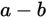• B.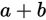• C.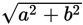• D.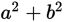Explanation:

C

Difficulty: Medium

Category: Additional Topics in Math / Geometry

Strategic Advice: You could use algebra to answer a question like this, or you could draw a quick sketch and use geometry. Use whichever method gets you to the answer quicker.

Getting to the Answer: Algebraic solution: The equation of a circle centered at the origin is given by the equation x2 + y2 = r2, where x and y represent any ordered pair (point) that lies on the circumference of the circle, and r is the length of the radius. Substitute the point (a, b) for x and y, and then solve for r: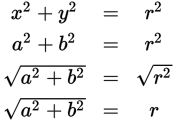This matches (C).

Geometric solution: Draw a sketch of a circle with center (0, 0). Add point (a, b) to the circle and draw in a radius to that point. Use the coordinates of the point to draw and label a right triangle. Your sketch should look like the following: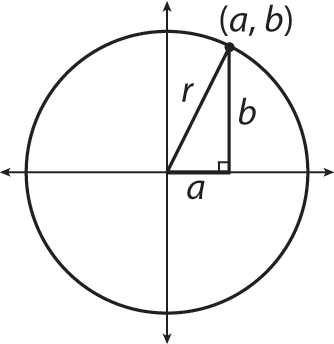Now use the Pythagorean theorem to find that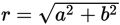, which agrees with the algebraic solution.